﻿ Cavity flow (mapping data to different mesh) - XSim

# Cavity flow (mapping data to different mesh)

OpenFOAM 4.x

## Summary

To run this case, you need to run the case "cavity (cavity flow)" at first.

After running the case "cavity", the following command must be executed in the parent directory of "cavity" to create and run the case "cavityFine".

mkdir cavityFine
cd cavityFine
cp -r ../cavity/0 .
cp -r ../cavity/system .
cp -r ../cavity/constant .
sed -i -e s/"20 20 1"/"41 41 1"/g system/blockMeshDict
sed -i -e s/"\(startTime[ \t]*\) 0;"/"\1 0.5;"/g system/controlDict
sed -i -e s/"\(endTime[ \t]*\) 0.5;"/"\1 0.7;"/g system/controlDict
sed -i -e s/"\(deltaT[ \t]*\) 0.005;"/"\1 0.0025;"/g system/controlDict
sed -i -e s/"\(writeControl[ \t]*\) timeStep;"/"\1 runTime;"/g system/controlDict
sed -i -e s/"\(writeInterval[ \t]*\) 20;"/"\1 0.1;"/g system/controlDict

blockMesh
mapFields ../cavity -sourceTime latestTime -consistent
icoFoam

The the analysis steps are as follows.

1. Calculate the flow up to 0.5 seconds in case "cavity".
2. Map the calculation results as initial conditions for the fine-mesh case "cavityFine".
3. Calculate the flow from 0.5 seconds to 0.7 seconds in case "cavityFine".
4. Map the results as initial conditions for the case "cavityGrade" (this tutorial), which has a coarse and fine mesh.
5. Calculate the flow from 0.5 seconds to 0.7 seconds in case "cavityGrade".

The boundary conditions are the same as case "cavity", as followsModel geometry

The meshes for "cavity", "cavityFine", and the case in this tutorial are shown below. They have a single mesh in the Z direction and will be calculated as a 2-dimensional problem.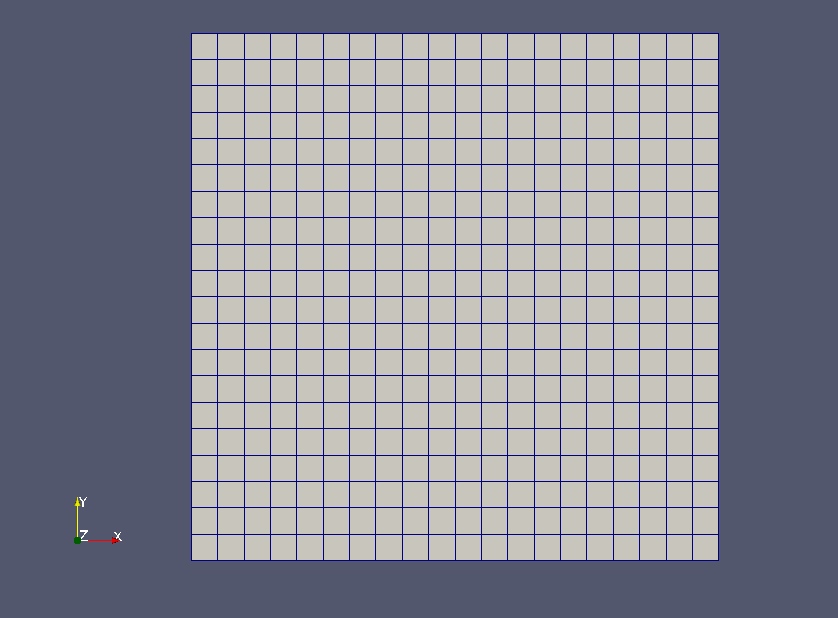cavity's mesh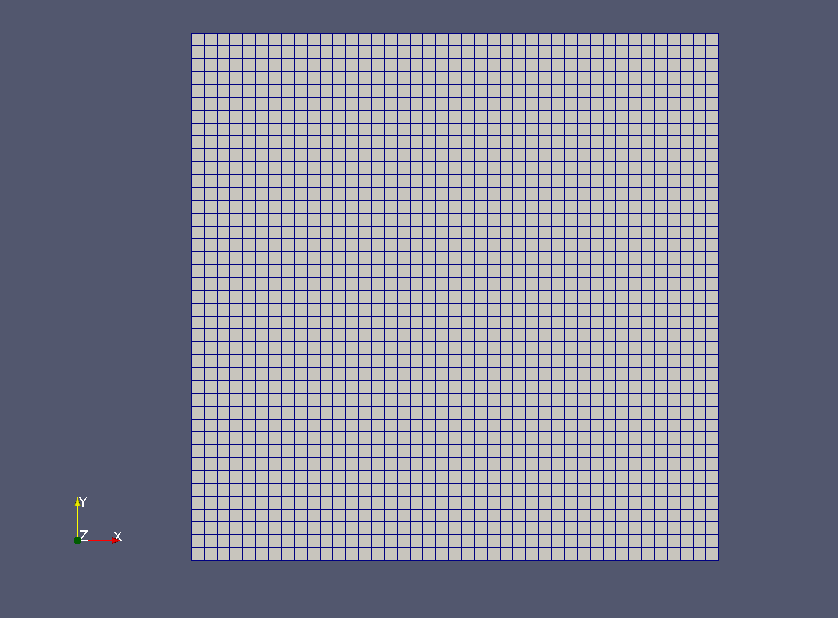cavityFine's mesh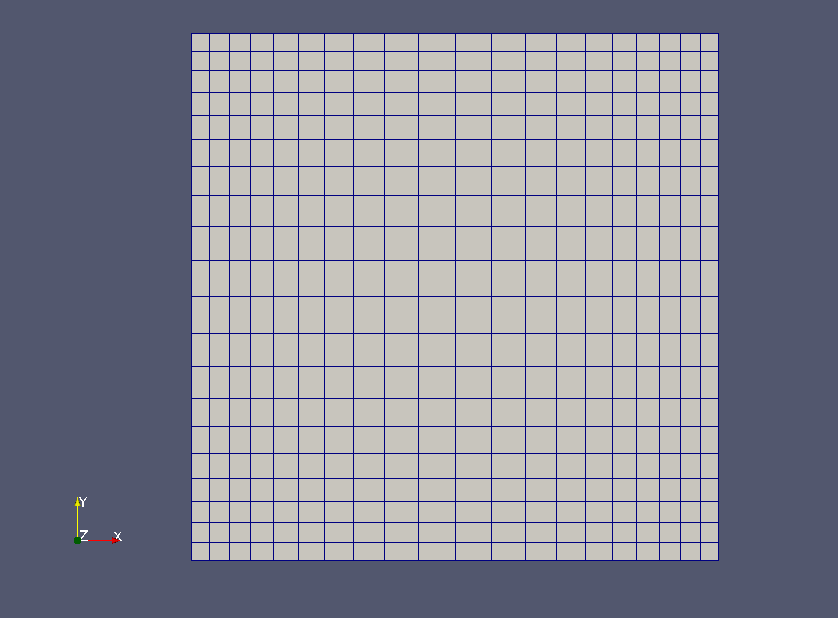The mesh in this tutorial

The calculation result of the case "cavityFine" will be mapped to the directory with mapFields, and be used as initial conditions.

The calculation result is as follows.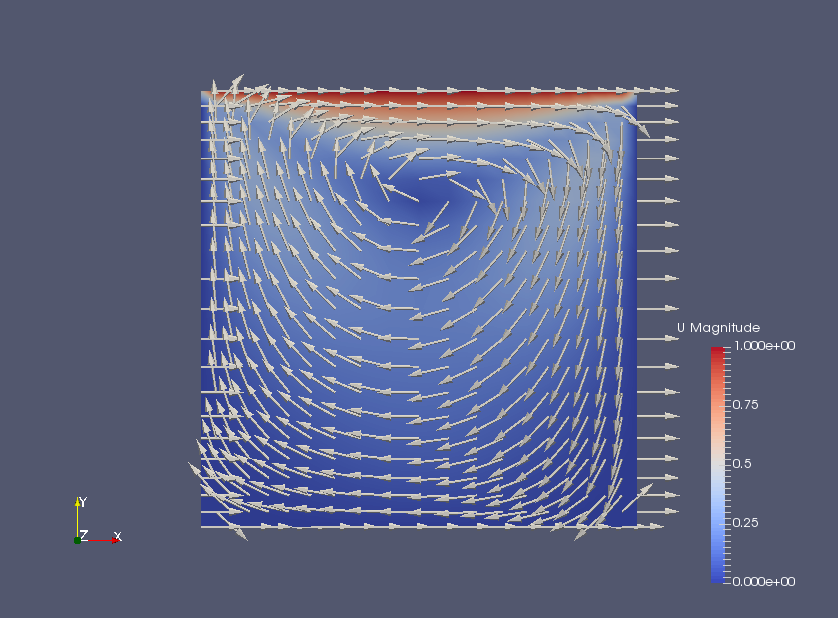Flow velocity at 0.7 seconds (U)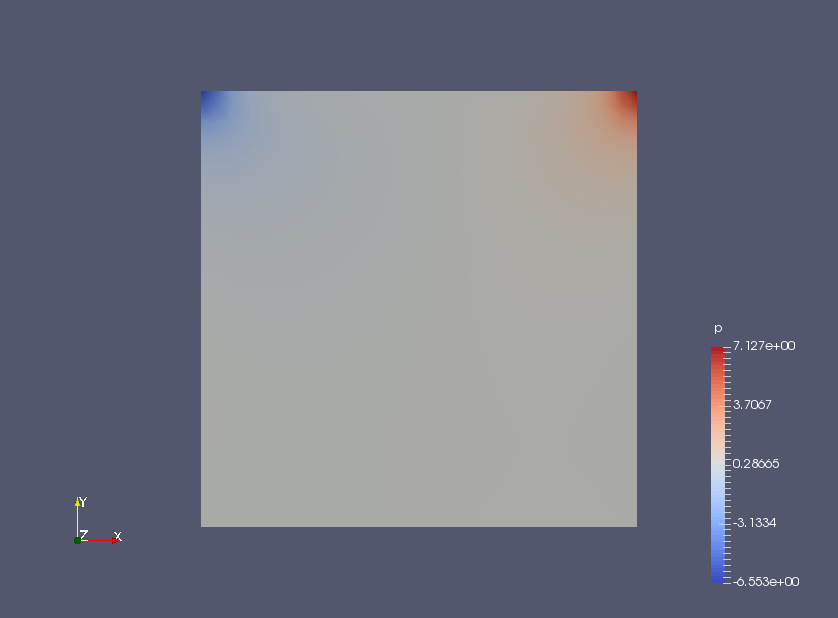Pressure at 0.7 seconds (p)

## Commands

blockMesh
mapFields ../cavityFine -sourceTime latestTime -consistent
icoFoam

paraFoam

The calculated cavityFine directory is assumed to be in the same directory which cavityGrade exists in.

If the mapping source and destination have the same geometry and boundary conditions, specify the option -consistent when running mapFields.

## Calculation time

--- *Single, Core(TM) i7-2600 CPU @ 3.40GHz 3.40GHz